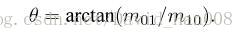# 图像处理检测质心

## 图像处理之计算二值连通区域的质心

2013-12-27 00:15:14 jia20003 阅读数 25504
• ###### 图像特征描述

掌握常用的图像边缘检测算子，及Canny算子基本原理 掌握灰度阈值化及大津算法； 掌握常见的图像特征描述方式 了解局部阈值分割、区域生长、分水岭算法及基于轮廓的阈值分割； 通过米粒检测实例掌握图像预处理、分割...

2622人学习 CSDN就业班
免费试看

1. Image Moments是图像处理中非常有用的算法，可以用来计算区域图像2. 什么是质心1.      输入图像转换为二值图像

2.      通过连通组件标记算法找到所有的连通区域，并分别标记

3.      对每个连通区域运用计算几何距算法得到质心

4.      用不同颜色绘制连通区域与质心，输出处理后图像1.      计算几何距算法代码

doublem00 = moments(pixels, width, height, 0, 0);

doublexCr = moments(pixels, width, height, 1, 0) / m00;// row

doubleyCr = moments(pixels, width, height, 0, 1) / m00;// column

return new double[]{xCr, yCr};

2.      连通组件标记算法代码参见这里:

http://blog.csdn.net/jia20003/article/details/7628371

```package com.gloomyfish.image.moments;

import java.awt.image.BufferedImage;

import com.gloomyfish.filter.study.AbstractBufferedImageOp;
import com.gloomyfish.rice.analysis.FastConnectedComponentLabelAlg;
// Geometric Moments Computing
// low-order moments - calculate the center point
// second-order moments - get angle size
// projection -
public class GeometricMomentsFilter extends AbstractBufferedImageOp {

@Override
public BufferedImage filter(BufferedImage src, BufferedImage dest) {
int width = src.getWidth();
int height = src.getHeight();

if ( dest == null )
dest = createCompatibleDestImage( src, null );

// first step - make it as binary image output pixel
int[] inPixels = new int[width*height];
int[] outPixels = new int[width*height];
getRGB( src, 0, 0, width, height, inPixels );
int index = 0;
for(int row=0; row<height; row++) {
int tr = 0;
for(int col=0; col<width; col++) {
index = row * width + col;
tr = (inPixels[index] >> 16) & 0xff;
if(tr > 127)
{
outPixels[index] = 1;
}
else
{
outPixels[index] = 0;
}
}
}

// second step, connected component labeling algorithm
FastConnectedComponentLabelAlg ccLabelAlg = new FastConnectedComponentLabelAlg();
ccLabelAlg.setBgColor(0);
int[] labels = ccLabelAlg.doLabel(outPixels, width, height);
int max = 0;
for(int i=0; i<labels.length; i++)
{
if(max < labels[i])
{
System.out.println("Label Index = " + labels[i]);
max = labels[i];
}
}

// third step, calculate center point of each region area(connected component)
int[] input = new int[labels.length];
GeometricMomentsAlg momentsAlg = new GeometricMomentsAlg();
momentsAlg.setBACKGROUND(0);
double[][] labelCenterPos = new double[max];
for(int i=1; i<=max; i++)
{
for(int p=0; p<input.length; p++)
{
if(labels[p] == i)
{
input[p] = labels[p];
}
else
{
input[p] = 0;
}
}
labelCenterPos[i-1] = momentsAlg.getGeometricCenterCoordinate(input, width, height);
}

// render the each connected component center position
for(int row=0; row<height; row++) {
for(int col=0; col<width; col++) {
index = row * width + col;
if(labels[index] == 0)
{
outPixels[index] = (255 << 24) | (0 << 16) | (0 << 8) | 0; // make it as black for background
}
else
{
outPixels[index] = (255 << 24) | (0 << 16) | (0 << 8) | 100; // make it as blue for each region area
}
}
}

// make it as white color for each center position
for(int i=0; i<max; i++)
{
int crow = (int)labelCenterPos[i];
int ccol = (int)labelCenterPos[i];
index = crow * width + ccol;
outPixels[index] = (255 << 24) | (255 << 16) | (255 << 8) | 255;
}

setRGB( dest, 0, 0, width, height, outPixels );
return dest;
}

}
```

Moment算法代码：

```package com.gloomyfish.image.moments;

public class GeometricMomentsAlg {
private int BACKGROUND = 0; // background color
private int labelIndex = 1;

public GeometricMomentsAlg()
{
System.out.println("Geometric Moments Algorithm Initialziation...");
}

public int getLabelIndex() {
return labelIndex;
}

public void setLabelIndex(int labelIndex) {
this.labelIndex = labelIndex;
}

public int getBACKGROUND() {
return BACKGROUND;
}

public void setBACKGROUND(int bACKGROUND) {
BACKGROUND = bACKGROUND;
}

public double[] getGeometricCenterCoordinate(int[] pixels, int width, int height)
{
double m00 = moments(pixels, width, height, 0, 0);
double xCr = moments(pixels, width, height, 1, 0) / m00; // row
double yCr = moments(pixels, width, height, 0, 1) / m00; // column
return new double[]{xCr, yCr};
}

public double moments(int[] pixels, int width, int height, int p, int q)
{
double mpq = 0.0;
int index = 0;
for(int row=0; row<height; row++)
{
for(int col=0; col<width; col++)
{
index = row * width + col;
if(pixels[index] == BACKGROUND) continue;
mpq += Math.pow(row, p) * Math.pow(col, q);
}
}
return mpq;
}

public double centralMoments(int[] pixel, int width, int height, int p, int q)
{
double m00 = moments(pixel, width, height, 0, 0);
double xCr = moments(pixel, width, height, 1, 0) / m00;
double yCr = moments(pixel, width, height, 0, 1) / m00;
double cMpq = 0.0;
int index = 0;
for(int row=0; row<height; row++)
{
for(int col=0; col<width; col++)
{
index = row * width + col;
if(pixel[index] == BACKGROUND) continue;
cMpq += Math.pow(row - xCr, p) * Math.pow(col - yCr, q);
}
}
return cMpq;
}

public double normalCentralMoments(int[] pixel, int width, int height, int p, int q)
{
double m00 = moments(pixel, width, height, 0, 0);
double normal = Math.pow(m00, ((double)(p+q+2))/2.0d);
return centralMoments(pixel, width, height, p, q)/normal;
}
}
```

## 基于灰度图像的质心算法和三角测距原理（学习笔记）

2019-01-10 18:12:14 walkmaner 阅读数 4537
• ###### 图像特征描述

掌握常用的图像边缘检测算子，及Canny算子基本原理 掌握灰度阈值化及大津算法； 掌握常见的图像特征描述方式 了解局部阈值分割、区域生长、分水岭算法及基于轮廓的阈值分割； 通过米粒检测实例掌握图像预处理、分割...

2622人学习 CSDN就业班
免费试看

（1）普通质心算法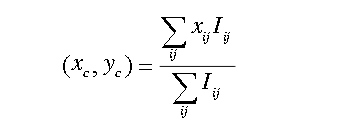（2） 强加权质心算法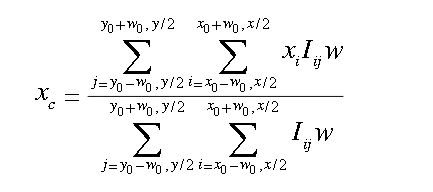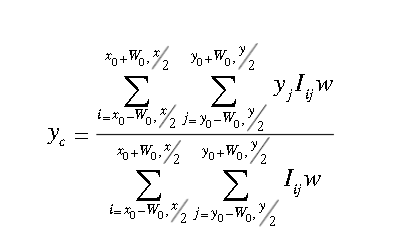其原理是将光斑中心较近的部分区域像素值增强，使得对光斑影响较大点的影响力进一步加大，提高质心探测精度，即使光斑形状不近似高斯分布，该方法依然能较准确的探测光斑质心。

（3）阈值质心算法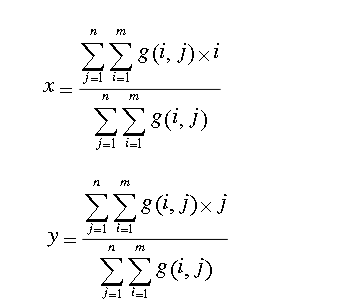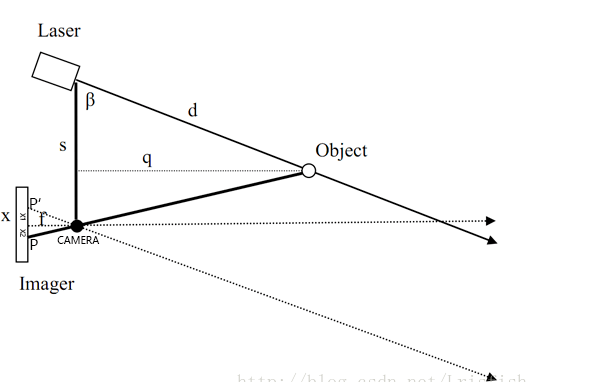设 PP′=x。则由相似三角形可得：

f/x=q/s  ==>  q=fs/x

其中pixelSize是像素单位大小， position是成像的像素坐标相对于成像中心的位置。

最后，可求得距离d:                       d=q/sin⁡β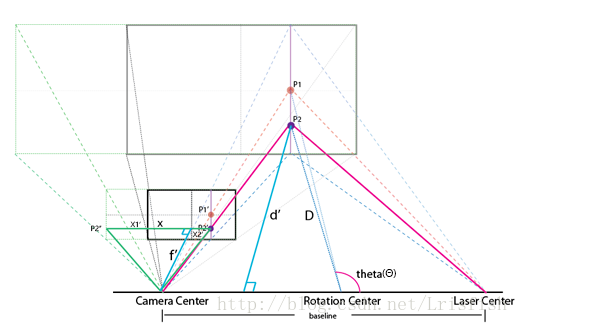由相似三角形原理：

d'/baseline=f'/x

d′是P2与baseline所成平面上P2到底边的高（类比于单点激光测距原理中的q）。同样x可分为两部分计算d′是P2与baseline所成平面上P2到底边的高（类比于单点激光测距原理中的q）。

f'/f=cos⁡θ

tan⁡θ=(|P2'.y-P1'.y|)/f

f'=f/cos⁡(arctan⁡((P2'.y-P1'.y)/f))

https://wenku.baidu.com/view/d1529dbef121dd36a32d8272.html?sxts=1547103591980

https://blog.csdn.net/Lrisfish/article/details/77838690?utm_source=copy

## 【matlab】:matlab求图像质心算法

2016-03-22 16:26:09 qq_23100787 阅读数 10653
• ###### 图像特征描述

掌握常用的图像边缘检测算子，及Canny算子基本原理 掌握灰度阈值化及大津算法； 掌握常见的图像特征描述方式 了解局部阈值分割、区域生长、分水岭算法及基于轮廓的阈值分割； 通过米粒检测实例掌握图像预处理、分割...

2622人学习 CSDN就业班
免费试看``````function y = centroid()
[M,N,P] = size(I);
int8 sumx ;
int8 sumy ;
int8 count ;
sumx = 0;
sumy = 0;
count = 0;
int8 R ;
int8 G ;
int8 B ;
R = 20; G = 200;B = 200;
for i = 1:1:M
for j = 1:1:N
if I(i,j,1)>=R &&  I(i,j,2)>=G && I(i,j,3)>=B
sumx = sumx + i;
sumy = sumy +j;
count = count + 1;
end
end
end
sumx = sumx / count;    %sumx存储了x轴坐标
sumy = sumy / count;    %sumy存储了y轴坐标
disp(sumx);disp(sumy);``````## 图形图像基本处理之——一个非常容易理解的图像求质心代码

2012-12-16 13:06:34 zhufanqie 阅读数 23065
• ###### 图像特征描述

掌握常用的图像边缘检测算子，及Canny算子基本原理 掌握灰度阈值化及大津算法； 掌握常见的图像特征描述方式 了解局部阈值分割、区域生长、分水岭算法及基于轮廓的阈值分割； 通过米粒检测实例掌握图像预处理、分割...

2622人学习 CSDN就业班
免费试看

下面贴出我的一个很简单且很容易理解的质心标志代码出来，供大家参考，代码都写了注释的，详情如下：

```clear;clc;close all;
figure(1);imshow(I);%显示原图像

I_gray=rgb2gray(I);%原图像变为灰度图像
level=graythresh(I_gray);%计算图像I_gray的全局阈值，level为标准化灰度值，其范围为[0 1]
[height,width]=size(I_gray);%计算灰度图像的长宽
I_bw=im2bw(I_gray,level);%im2bw使用阈值level将灰度图像转换为二值图像．
figure(2);imshow(I_bw);%显示二值图像（背景为白色）

for i=1:height %%循环中进行反色
for j=1:width
if I_bw(i,j)==1
I_bw(i,j)=0;
else I_bw(i,j)=1;
end
end
end
figure(3);imshow(I_bw);%显示取反后的二值图像（背景为黑色）

[L,num]=bwlabel(I_bw,8);%bwlabel标注二值图像I_bw中的目标物体，返回标识矩阵Ｌ和I_bw中目标物体的数量num，８表示连通数．
plot_x=zeros(1,num);%%zeros(m,n)产生m×n的全0矩阵．用于记录质心位置的横坐标
plot_y=zeros(1,num);%zeros(m,n)产生m×n的全0矩阵．用于记录质心位置的纵坐标

for k=1:num  %%num个区域依次统计质心位置
sum_x=0;    sum_y=0;    area=0; %初始化
for i=1:height
for j=1:width
if L(i,j)==k
sum_x=sum_x+i;  %计算第Ｋ区域的横坐标总和
sum_y=sum_y+j;  %计算第Ｋ区域的纵坐标总和
area=area+1;    %计算第Ｋ区域的由多少个坐标点表示
end
end
end
plot_x(k)=fix(sum_x/area);  %计算第Ｋ区域的质心横坐标
plot_y(k)=fix(sum_y/area);%计算第Ｋ区域的质心纵坐标
end

figure(4);imshow(I_bw);%显示取反后的二值图像（背景为黑色）,并在图上标注质心点位置
for i=1:num
hold  on
plot(plot_y(i) ,plot_x(i), '*')
end```从上面的图片看来，此方法对子区域较明显的图片有较强的分辨率，但是针对图片的子区域不那么的显著地情况呢，下面我们用另一幅图片进行测试，运行结果如下：所以对图片特定区域求质心还有待进一步研究。

## 数字图像处理学习笔记（一）：特征检测和匹配概述

2019-09-23 17:15:53 Vichael_Chan 阅读数 452
• ###### 图像特征描述

掌握常用的图像边缘检测算子，及Canny算子基本原理 掌握灰度阈值化及大津算法； 掌握常见的图像特征描述方式 了解局部阈值分割、区域生长、分水岭算法及基于轮廓的阈值分割； 通过米粒检测实例掌握图像预处理、分割...

2622人学习 CSDN就业班
免费试看

## 1、特征点概述

• 关键点（Keypoint）：关键点指的是该特征点在图像中的位置，有些还具有方向、尺度信息；
• 描述子（Descriptor）：描述子通常是一个向量，按照人为的设计的方式，描述关键点周围像素的信息
通常描述子是按照外观相似的特征应该有相似的描述子设计的。因此，在匹配的时候，只要两个特征点的描述子在向量空间的距离相近，就可以认为它们是同一个特征点。

## 2、特征点的匹配

1）提取图像中的关键点，这部分是查找图像中具有某些特征（不同的算法有不同的）的像素；
2）根据得到的关键点位置，计算特征点的描述子；
3）根据特征点的描述子，进行匹配；

## 3、特征点描述子

• 不变性：指特征不会随着图像的放大缩小旋转而改变；
• 鲁棒性：对噪声、光照或者其他一些小的形变不敏感；
• 可区分性：每一个特征描述子都是独特的，具有排他性，尽可能减少彼此间的相似性。

• 尺度不变性 (Scale Invarient)
指的是同一个特征，在图像的不同的尺度空间保持不变。匹配在不同图像中的同一个特征点经常会有图像的尺度问题，不同尺度的图像中特征点的距离变得不同，物体的尺寸变得不同，而仅仅改变特征点的大小就有可能造成强度不匹配。如果描述子无法保证尺度不变性，那么同一个特征点在放大或者缩小的图像间，就不能很好的匹配。为了保持尺度的不变性，在计算特征点的描述子的时候，通常将图像变换到统一的尺度空间，再加上尺度因子
• 旋转不变性 (Rotation Invarient)
指的是同一个特征，在成像视角旋转后，特征仍然能够保持不变。和尺度不变性类似，为了保持旋转不变性，在计算特征点描述子的时候，要加上关键点的方向信息。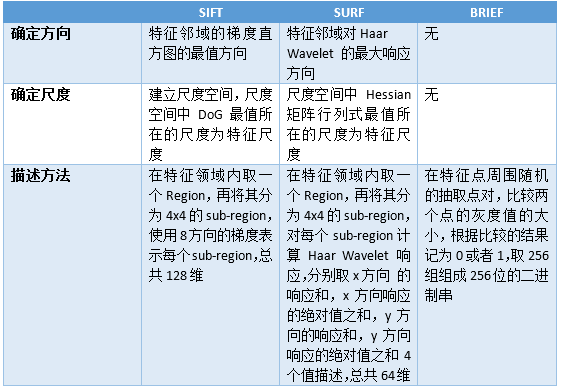## 4、常用的特征点算法

• 特征点的提取，在图像检测到特征点的位置。
• 特征点的描述，也就是描述子。

### 一、SIFT

SIFT算法主要有以下几个步骤：

• 1）高斯差分金字塔的构建
使用组和层的结构构建了一个具有线性关系的金字塔（尺度空间），这样可以在连续的高斯核尺度上查找图像的特征点；另外，它使用一阶的高斯差分来近似高斯的拉普拉斯核，大大的减少了运算量。
• 2)尺度空间的极值检测及特征点的定位
搜索上一步建立的高斯尺度空间，通过高斯差分来识别潜在的对尺度和旋转不变的特征点。但是，在离散空间中，局部极值点可能并不是真正意义的极值点，真正的极值点有可能落在离散点的间隙中，SIFT通过尺度空间DoG函数进行曲线拟合寻找极值点
特征方向赋值
• 3)基于图像局部的梯度方向，分配给每个关键点位置一个或多个方向，后续的所有操作都是对于关键点的方向、尺度和位置进行变换，从而提供这些特征的不变性。
• 4)特征描述子的生成
通过上面的步骤已经找到的SIFT特征点的位置、方向、尺度信息，最后使用一组向量来描述特征点及其周围邻域像素的信息。

SIFT算法中及包含了特征点的提取算法，也有如何生成描述子的算法，更进一步的SIFT算法介绍可参看：

### 二、SURF

SURF全称 Speeded Up Robust Features “加速版的具有鲁棒性的特征”，是在SIFT算法的基础上提出的，主要针对SIFT算法运算速度慢，计算量大的缺点进行了改进。
SURF的流程和SIFT比较类似，这些改进体现在以下几个方面：

• 1）特征点检测是基于Hessian矩阵，依据Hessian矩阵行列式的极值来定位特征点的位置。并且将Hession特征计算与高斯平滑结合在一起，两个操作通过近似处理得到一个核模板。
• 2）在构建尺度空间时，使用box filter与源图像卷积，而不是使用DoG算子。
• 3）SURF使用一阶Haar小波在x、y两个方向的响应作为构建特征向量的分布信息。

### 三、FAST特征点提取算法

SIFT和SURF是非常好的，稳定的特征点算法，但运算速度是其一大弊端，无法做到实时的特征提取和匹配，其应用就有了很大的局限性。FAST特征提取算法弥补了这一局限，检测局部像素灰度变化明显的地方，以速度快而著称，其全称为：Features From Accelerated Segment Test。在FAST算法的思想很简单：如果一个像素与周围邻域的像素差别较大（过亮或者过暗），那么可以认为该像素是一个角点。和其他的特征点提取算法相比，FAST算法只需要比较像素和其邻域像素的灰度值大小，十分便捷。

FAST算法提取角点的步骤：

• 1）在图像中选择像素p，假设其灰度值为：Ip
• 2）设置一个阈值T，例如：Ip 的20%
• 3）选择p周围半径为3的圆上的16个像素，作为比较像素
• 4）假设选取的圆上有连续的N个像素大于 Ip+T 或者 Ip-T，那么可以认为像素p就是一个特征点。
（N通常取12，即为FAST-12；常用的还有FAST-9,FAST-11）。

FAST算法只检测像素的灰度值，其运算速度极快，同时不可避免的也有一些缺点：

• 检测到的特征点过多并且会出现“扎堆”的现象。这可以在第一遍检测完成后，使用非最大值抑制（Non-maximal suppression），在一定区域内仅保留响应极大值的角点，避免角点集中的情况。
• FAST提取到的角点没有方向和尺度信息

BRIEF描述子

BRIEF是一种二进制的描述子，其描述向量是0和1表示的二进制串。0和1表示特征点邻域内两个像素（p和q）灰度值的大小：如果p比q大则选择1，反正就取0。在特征点的周围选择128对这样的p和q的像素对，就得到了128维由0，1组成的向量。那么p和q的像素对是怎么选择的呢？通常都是按照某种概率来随机的挑选像素对的位置。

BRIEF使用随机选点的比较，速度很快，而且使用二进制串表示最终生成的描述子向量，在存储以及用于匹配的比较时都是非常方便的，其和FAST的搭配起来可以组成非常快速的特征点提取和描述算法。

### 四、ORB算法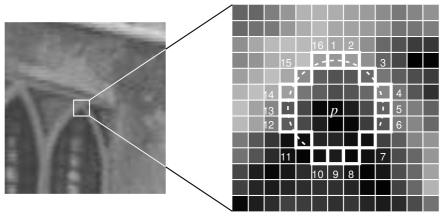ORB的全称是Oriented FAST and Rotated BRIEF，是目前来说非常好的能够进行的实时的图像特征提取和描述的算法，它改进了FAST特征提取算法，并使用速度极快的二进制描述子BRIEF。

• 使用非最大值抑制，在一定区域内仅仅保留响应极大值的角点，避免FAST提取到的角点过于集中。
• FAST提取到的角点数量过多且不是很稳定，ORB中可以指定需要提取到的角点的数量N，然后对FAST提取到的角点分别计算Harris响应值，选择前N个具有最大响应值的角点作为最终提取到的特征点集合。
• FAST提取到的角点不具有尺度信息，在ORB中使用图像金字塔，并且在每一层金字塔上检测角点，以此来保持尺度的不变性。
• FAST提取到的角点不具有方向信息，在ORB中使用灰度质心法(Intensity Centroid)来保持特征的旋转不变性。

FAST-12算法：

• 问题1：FAST特征点的数量很多，并且不是确定，而大多数情况下，我们希望能够固定特征点的数量。

• 问题2：FAST角点不具有方向信息和尺度问题。

1）在一个小的图像块B中，定义图像块的矩为：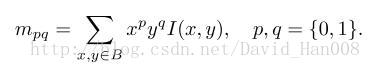2）通过矩找到图像块的质心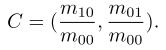3）连接图像块的几何中心o与质心C，得到一个oc的向量，把这个向量的方向定义特征点的方向